© 2021 - First Crazy Developer (Abhishek Kumar)

###Stack & Heap - Behind the scene in .Net Framework

One of the most essential parts of coding in the .NET Framework is "Variable Declaration".  In order to understand the importance of this term, we’ll need to explore a few other terms which are fundamental to .NET, and to some extent, programming in general.  So, in the next few articles, we’ll be discussing:

"Stack & Heap", "Value types & Reference types" and "Boxing & Unboxing".

The three terms above are related to each other.  If we’re planning on talking about Variable Declaration, at the very least we need to know the core concepts of those terms.  They all point to a couple of important questions:  “What goes into a variable once we declare it?” and “What happens to that variable when we’re done with it?”

First of all, what happens in our .NET application when we declare a variable?  Surely even a novice programmer knows that it allocates a chunk of memory to the RAM.

This memory has three important features:

1. The NAME of the variable
2. The DATA TYPE of the variable
3. The VALUE of the variable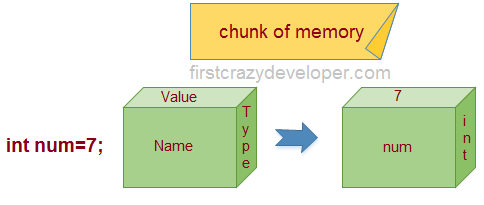Now we know what information is held in memory.  Simple right?  Well, not quite.  Depending on how the variable is defined, different types of memory allocation take place in .NET FrameWork.  Those two types of allocation are:

1. STACK memory allocation
2. HEAP memory allocation

In order to understand Stack and Heap, let's take a look at the following code:

1. static void Main(string[] args)
2. {
3. int num1 = 7;
4. int num2 = 16;
5. Bike bikeObj = new Bike();
6. }

Here we’ve simply added three lines of code to our Main method.  Let’s discuss and understand line by line how things execute internally.

Line 1:  When we execute this line, the compiler allocates a small amount of memory in the Stack.  Here, the Stack is responsible for keeping track of the running memory needed in our application.

Line 3:  The Compiler moves to the next step.  It “stacks” this memory allocation on top of the first one – hence the name STACK.

Stack is a series of compartments or boxes put on top of each other. This allocation and de-allocation is done using LIFO (Last In First Out) logic.  Simply put, memory is allocated and de-allocated at only one end of the memory (the top of the Stack).

Line 5:  In this line, we define an object.  Here, the process of memory allocation is different.  What we’ve done is created a reference pointer on the Stack, but the actual object is stored in a different type of memory called the Heap. The Heap doesn't track running memory, it's just a pile of objects which can be reached at any moment of time.  It is used for 'dynamic memory allocation'.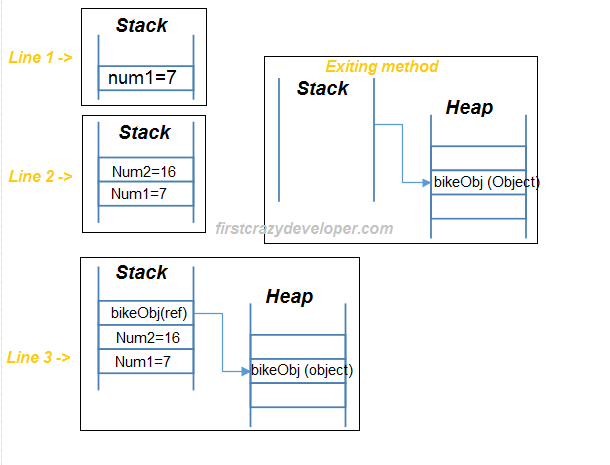So, we have some important things to note:

1. In the above example, reference pointers are allocated on the Stack.  If all we wrote was ‘Bike bikeObj’, that wouldn’t allocate memory for an instance of Bike; it would only allocate a Stack variable bikeObj with a value of null.  It doesn’t get allocated to the Heap until we assign it using the ‘new’ keyword.

2. At the end of our method, all memory variables assigned to the Stack are cleared.  That is, all variables which are allocated on the Stack are de-allocated in 'LIFO' fashion from the Stack. The one concerning point here is whether or not any of the memory on the Heap was de-allocated. This is where the Garbage Collector (GC) comes in.  You may be wondering…

Why two types of memory?  Can't we just allocate everything as one memory type and call it a day?   Do we really need a Garbage Collector?

In the example code above, we see that primitive data types are simple not complex.  They hold single values like int num1 = 7, int num2 = 16.  However, class object data types are complex.  They always reference other primitives or object data types.  Simply put, they hold a reference to other multiple values and must be stored in memory.  Object types need dynamic memory but primitive types need static memory.

To sum it all up:

If the requirement is for dynamic memory, it's allocated on the Heap or else it goes on the Stack.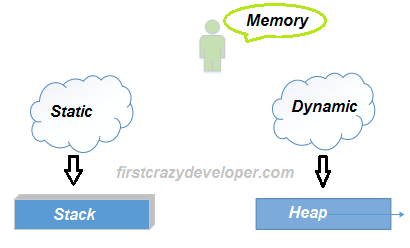I hope we’ve made some progress with Stack and Heap in .NET. Let’s take a look at some other examples to further our understanding of the concepts.

Take a look at the following code:

1. public int SampleMethod(int num)
2. {
3. int result;
4. result = num + 7;
5. return result;
6. }

We see there’s a simple method named "SampleMethod" with a normal integer type parameter named "num".  You can imagine what happens once we start executing the method.  The method's parameters are placed on the Stack.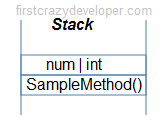Note: The method does not live on the stack and is illustrated just for reference.

When we execute the above code, ‘JIT compilation’ is performed if this is the first time we are hitting the method.  When the method executes, we need some more memory for the "result" variable which is allocated on the Stack.

Look at the following image: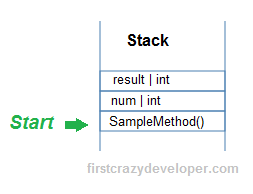Note: The method does not live on the stack and is illustrated just for reference.

Above "SampleMethod" finishes execution and our result is returned.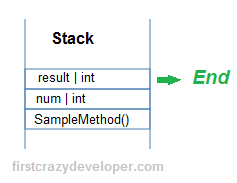Note: The method does not live on the stack and is illustrated just for reference.

After the method "SampleMethod" exits, all the occupied memory in the Stack is de-allocated from RAM.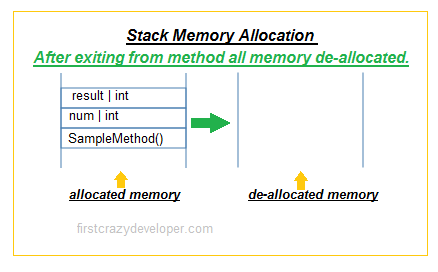In the above given example we can see that whenever a primitive type variable is declared within the body of a method, it will be placed on the Stack.

Now, primitive type variable are also sometimes placed on the Heap.  Do you remember the rule that states that primitive types always go where they were declared?  Well, if a primitive type is declared outside of a method, but inside a complex object type, then it will be placed on the Heap.

Look at the following code:

If we have the following "MathSample" class (which is a complex type because it is a class):

1. public class MathSample
2. {
3.    public int result;
4. }

and also we have the following method:

1. public MathSample SampleMethod(int num)
2. {
3.     MathSample sampleObj = new MathSample ();
4.      sampleObj.result = num + 5;
5.      return sampleObj;
6. }

When we execute the above method "SampleMethod", the method's parameters are placed on the Stack.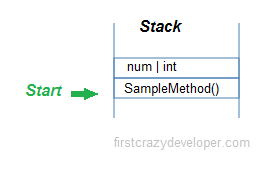Note: The method does not live on the stack and is illustrated just for reference.

Now when the pointer is moved to the first line of the method, it gets interesting. Because "MathSample" is a complex type, it is placed on the Heap and referenced by a Pointer on the Stack.

Look at the following image: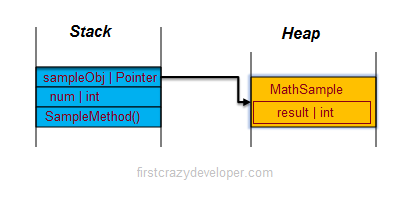Note: The method does not live on the stack and is illustrated just for reference.

After "SampleMethod" is finished executing (like in the above example), all the Stack memory is de-allocated from RAM but Heap memory still remains in RAM as an ‘orphan’. This is where the Garbage Collection (GC) comes into play to de-allocate this memory.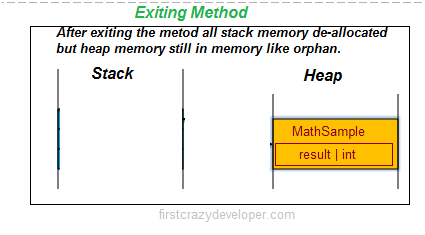I hope all of the above scenarios were enough for us to understand the basic concepts of Stack & Heap in the .NET Framework.  In a future post, we will take a deeper, more complex look at Stack & Heap.

Value and Reference Type

Moving on, we’ll discuss the concept of Value & Reference type in the .NET FrameworkValue types are types which hold both data and memory in the same location.  A Reference type has a pointer which points to the other memory location. Let’s understand what actually happens internally in the code below:

1. static void Main(string[] args)
2. {
3. int num1 = 7;
4. int num2 = num1;
5. }

We can see that there is a simple integer type variable named num1, whose value is assigned to another simple integer type variable named num2. Both of these memory values are allocated on the Stack.

When we assign the int value to the other int value, it creates a completely different copy. In other words, if you change one of them, the other does not change.  These kinds of data types are called ‘Value types’.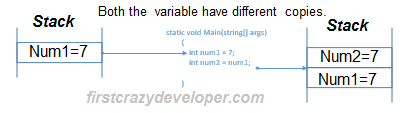When we create an object and when we assign an object to another object, both the created object and the assigned object point to the same memory location as shown in the code snippet below. So when we assign bikeObj to bikeObjDup, they both point to the same memory location. In other words if we change one of them, the other object is also affected.  This is termed as Reference types’.

1. static void Main(string[] args)
2. {
3. Bike bikeObj=new Bike();
4. Bike bikeObjDup = bikeObj;
5. }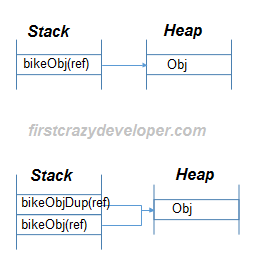So now a question comes to mind.  Which data types are ref types and which are value types?

In .NET depending on the data type, the variable is either assigned on the stack or on the heap. ‘String’ and ‘Objects’ are reference types, and any other .NET primitive data types are assigned to the Stack. Look at the image below for more details: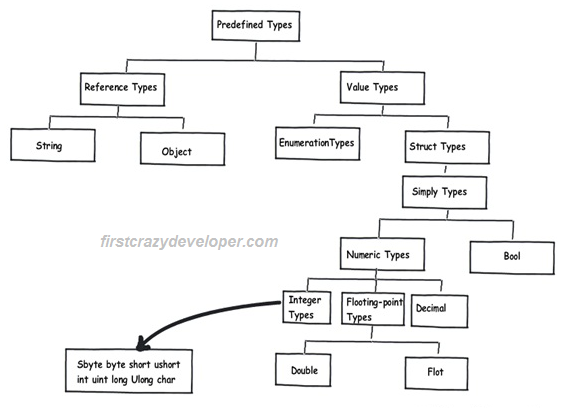Boxing and Unboxing

Up to this point we’ve discussed Stack, Heap, Value and Reference types in depth.  But the big question is how are those concepts actually used in day-to-day programming?

One of the biggest challenges is understanding the performance hit on our application, which is incurred due to data moving from the Stack to the Heap and vice versa.

Look at the following code:

1. public void SampleMethod()
2. {
3. int num=7;
4. object obj=num;
5. int num2=(int)obj;
6. }

There’s a method named "SampleMethod". In this method we defined an integer type variable named "num" and assigned some value to it.  In the next line we defined an object type variable named "obj" and assigned "num" to that.  We then defined one more integer type variable named "num2" and assigned "obj" to that.

In a nutshell, we can say the above example is the basis of a coupe of new concepts known as Boxing and Unboxing.  Simply defined, Boxing and Unboxing is the way through which we can move data from the stack to the heap and vice-versa.

Like in the above example, "num" is primitive type data which is allocated on the Stack.  We then defined "obj" (which is object type data allocated on the Heap).  Here, when we assigned the "num" into "obj", that process forces the movement of data from the Stack to the Heap.  In the next line we defined "num2" which is again primitive type data allocated on the Stack.  However, when we assigned obj into "num2" that process moves data from the Heap to the Stack.

In order to see how the performance is impacted, we ran the two functions 10,000 times. One function has Boxing and the other function is Simple. We used a stop watch object to monitor the time.

The Boxing function was executed in 3542 ms.  The function without Boxing was executed in 2477 ms.  There’s a clear difference in performance here.  In other words, try to avoid Boxing and Unboxing.  In a project where you need Boxing and Unboxing, use it when it’s absolutely necessary.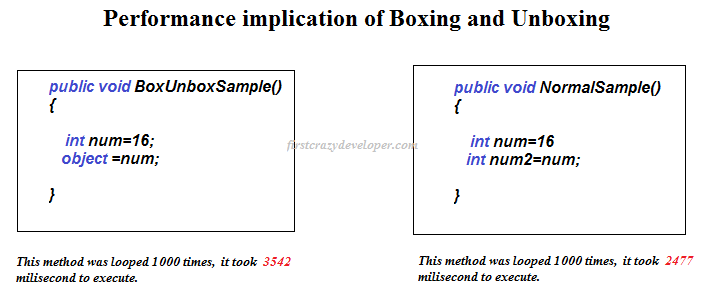Home Page 05 August 2015